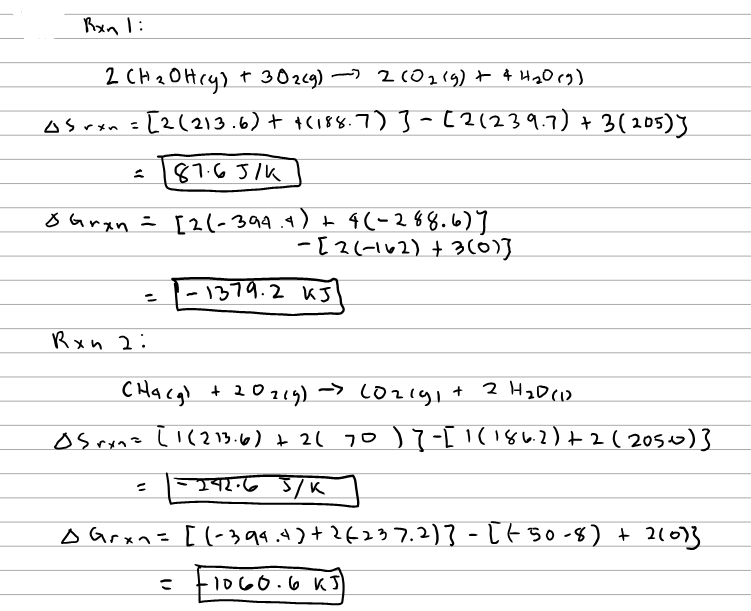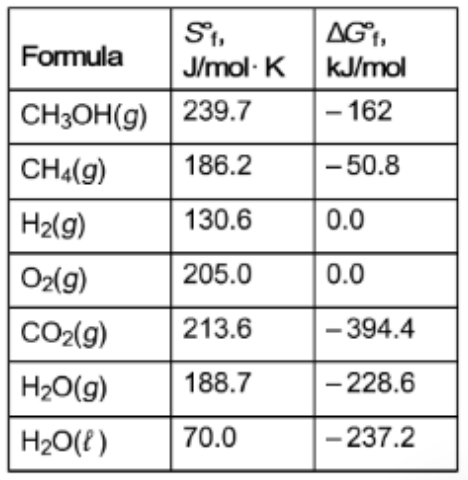# Problem: Rxn 1: 2CH3OH (g) + 3O2 (g) → 2CO2 (g) + 4H2O(g) ΔS°rxn =                                            ΔG°rxn = Rxn 2: CH4 (g) + 2O2 (g) → CO2 (g) + 2H2O(l) ΔS°rxn =                                            ΔG°rxn = Rxn 3: 2H2 (g) + O2 (g) → 2H2O(g) ΔS°rxn =                                            ΔG°rxn =

###### FREE Expert Solution
79% (321 ratings)
###### FREE Expert Solution79% (321 ratings)###### Problem Details

Rxn 1: 2CH3OH (g) + 3O2 (g) → 2CO2 (g) + 4H2O(g)

ΔS°rxn =                                            ΔG°rxn

Rxn 2: CH4 (g) + 2O2 (g) → CO2 (g) + 2H2O(l)

ΔS°rxn =                                            ΔG°rxn

Rxn 3: 2H2 (g) + O2 (g) → 2H2O(g)

ΔS°rxn =                                            ΔG°rxn =